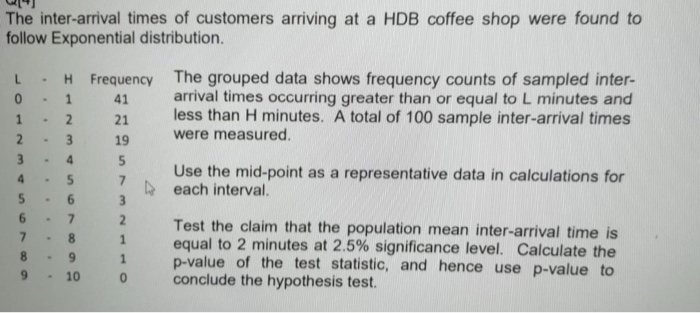# The inter-arrival times of customers arriving at a HDB coffee shop were found to follow Exponential...

###### Question:The inter-arrival times of customers arriving at a HDB coffee shop were found to follow Exponential distribution L H Frequency The grouped data shows frequency counts of sampled inter- 0 1 41 arrival times occurring greater than or equal to L minutes and 1 2 21 less than H minutes. A total of 100 sample inter-arrival times 23 19 were measured. Use the mid-point as a representative data in calculations for each interval. Test the claim that the population mean inter-arrival time is equal to 2 minutes at 2.5% significance level. Calculate the 8 9 1 p-value of the test statistic, and hence use p-value to 9 10 conclude the hypothesis test.